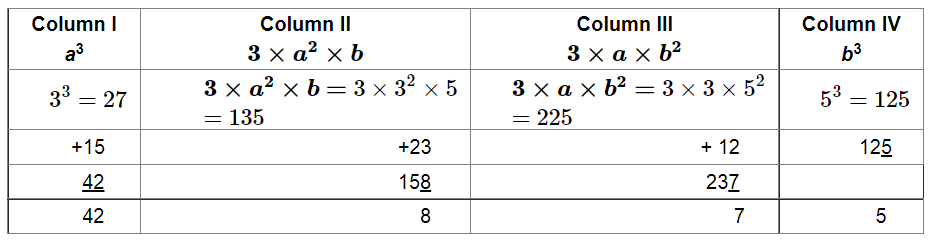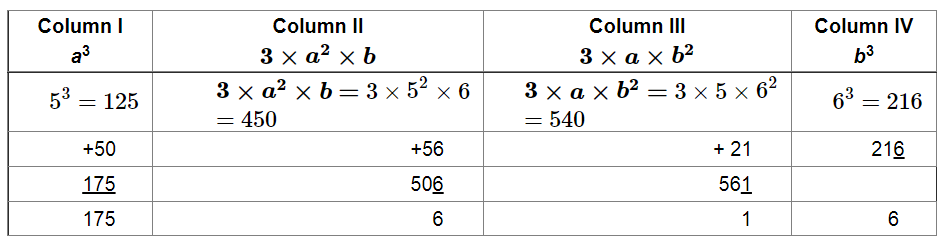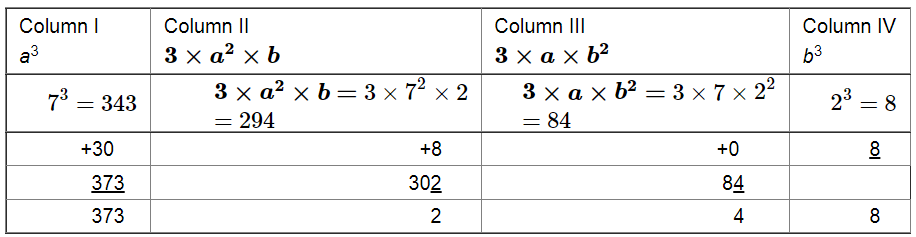# Find the cubes of the following numbers by column method:`
Question:

Find the cubes of the following numbers by column method:

(i) 35

(ii) 56

(iii) 72

Solution:

(i)

We have to find the cube of 35 using column method. We have: $a=3$ and $b=5$Thus, cube of 35 is 42875.

(ii)
We have to find the cube of 56 using column method. We have: $a=5$ and $b=6$Thus, cube of 56 is 175616.

(iii)
We have to find the cube of 72 using column method. We have: $a=7$ and $b=2$Thus, cube of 72 is 373248.